# Missing Data Imputation Approaches | How to handle missing values in Python

Machine Learning works on the idea of garbage in – garbage out. If you put in useless junk data to the machine learning algorithm, the results will also be, well, ‘junk’. The quality and consistency of results depend on the data provided. Missing values in data degrade the quality.

Why clean the data before training ML?

If you feed in bad data, expect bad results. Sometimes, you might even get lucky to get good results at certain a snapshot with junk data, but as you apply the model to predict on newer instances of data, the true performance shows up. It’s important to clean the data before training the model.

## The Problem with Raw data

One of the common problems with real raw data is, they often contain missing values. If you are going to be using ML algorithms from ‘scikit-learn’, these data need to be imputed, before they can be used.

Missing values in the data can occur for various reasons: There could be issues at the data collection stage, or they could occur systemically for some valid reason, or could just be a formatting issue with the database, programming language, systems compatibility, etc.

So, based on the reason, missing data is broadly classified into 3 broad types.

## Types of missing values

• Missing completely at random (MCAR): Probability of missing is the same across all the variables.

• Missing at random (MAR): Missing at random but it is possible to predict the missing value based on other variables.

• Not missing at random (NMAR): Can be handled by studying the root cause of missing

No need to remember this, just know the ideas.

So, What to do in case when missing values are Not Missing at Random (NMAR) ?

When it is NMAR, determine what the appropriate value should be when the values are missing and then apply that specific rule to impute the missing values.

For example, in sales datasets for spare parts, if certain there are certain dates missing, it could very well mean that there were no sales on those days. So, just replace such values with zero.

scikit-learn algorithms need missing value imputation but XGBoost, LightGBM etc do not require missing value imputation. Nevertheless, it’s a good practice to clean the data appropriately before use.

## Common reasons for missing data

1. Data might not be available for the complete time period of analysis
2. Non-occurence of events. for example, a student’s exam mark may be missing because he/she may not have taken the test.
3. Skipped response for some questions of the survey
4. Non-applicability of questions
5. Missing out at random

## What to do when the data is missing?

When there are missing values in data, you have four options:

• Approach 1: Drop the row that has missing values.
• Approach 2: Drop the entire column if most of the values in the column has missing values.
• Approach 3: Impute the missing data, that is, fill in the missing values with appropriate values.
• Approach 4: Use an ML algorithm that handles missing values on its own, internally.

Question: When to drop missing data vs when to impute them?

The common convention is, if you have large amount of data (rows) AND you will still be left with a large enough number of data points, representing the various different patterns and cases, that the ML model can learn from. Then it’s probably a good idea to drop the missing data points.

But, Why not impute them?

Because, filling in with data like the mean, median etc, is going to introduce some amount of approximation (incorrectness) to it. So if you have large volumes of data, you might wish to drop those records instead of introducing approximate values that could affect the ML model performance instead of enhancing it.

An exception of course is when you KNOW for certain what the data should be if the values are missing.

For example, if the data is about exam scores which contains missing values, because the candidates didn’t give the exams. In that case, you might want to replace the missing with 0.

IMPORTANT NOTE:

This is a part of the ‘Your First Machine Learning Project‘ series.

We will use the Churn modeling dataset_m and the following code blocks will continue where we left from the Exploratory Data Analysis (EDA) lesson.

To only import the dataset used in these the examples see ML Modeling lesson.

## Import the dataset

# Read data in form of a csv file download from above link.

# or

# First 5 rows of the dataset


## How to visualize pattern of missing values?

You can visualize missing values in the data using missingno package. It gives a very clear picture.

Let’s first install it by running pip install in terminal or anaconda prompt.

!pip install missingno

import missingno as msno # to visualize missing value


Visualize the presence of missing data. The matrix method will make a black bar for each column with the missing data points marked at various locations.

# visualize missing data
msno.matrix(df)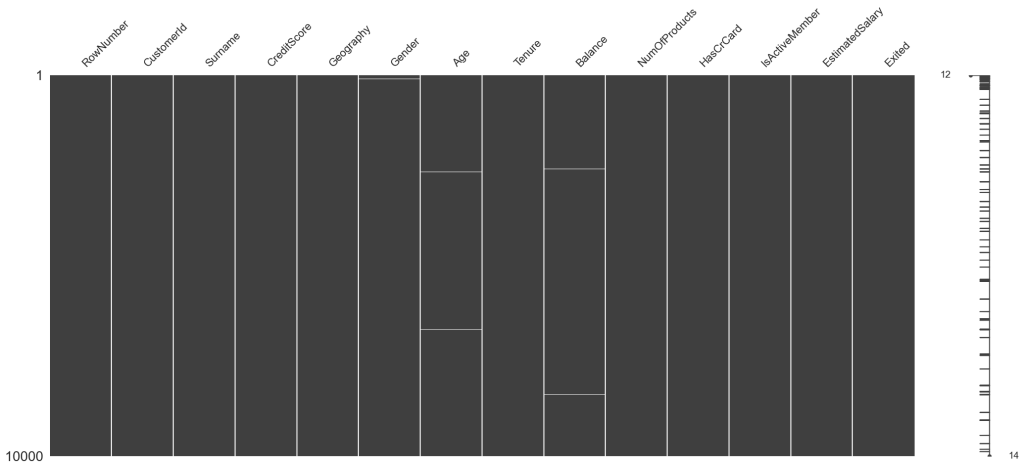## Do the missingness of values in one column affect the missing data behaviour in another?

Check the Correlation of missing values amongst columns

A high correlation would mean the missingness of values in one column is related to the missingness of values in another column. This can sometimes help gain more insights as to why two are more columns may be missing together and formulate a suitable tactic to handle it.

msno.heatmap(df)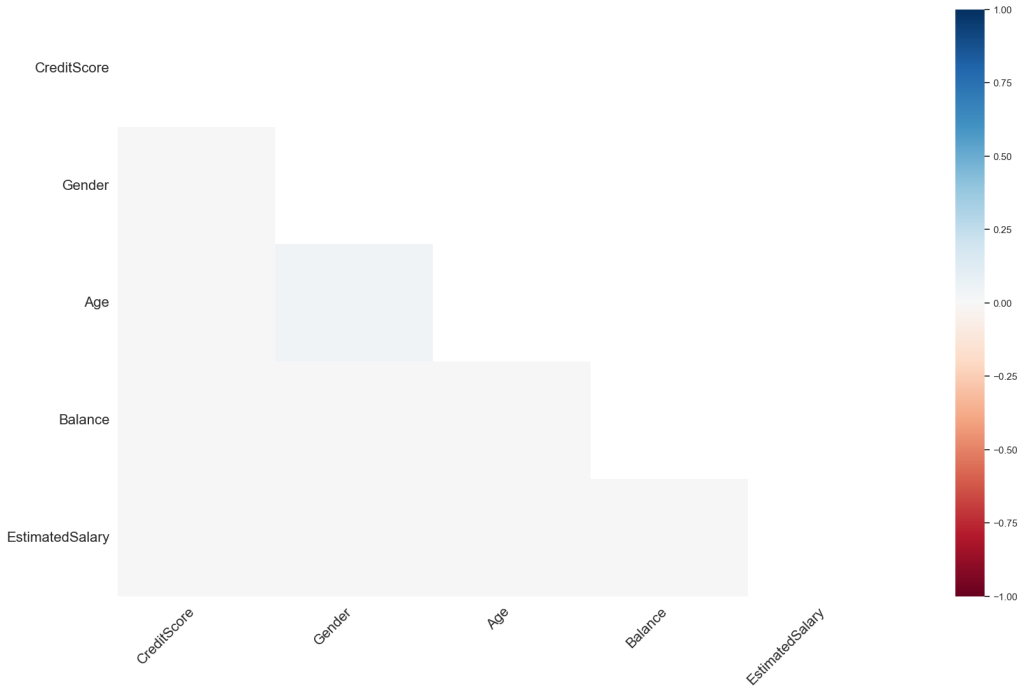## How to impute missing values?

When imputing with missing values, broadly you have two approaches.

1. Impute with a constant number
1. For numeric data:
• Mean of entire column excluding the missing values
• Median of entire column excluding the missing values
• Mean or median of only those rows (of the variable) that has the same value of Y as the missing.
2. For categorical data:
• Fill it with the most frequent data (mode)
2. Predict the missing value with machine learning using other non-missing columns as predictors.

Before we start imputing, let’s quickly look at the DataFrameSummary from DataTile to get a sense of the missing data

# Exhaustive Summary of dataframe
from datatile.summary.df import DataFrameSummary
dfs = DataFrameSummary(df)
dfs.columns_stats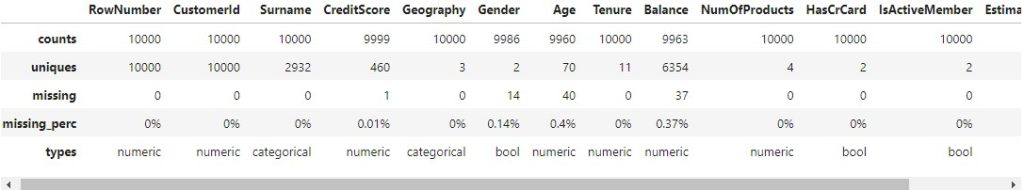## Approach 1: Dropping rows that contail missing values

Drop rows that contain missing values in Age

# Drop rows that contain missing values in AgeDrop rows that contain missing values in Age or Balance

df_app1 = df[(df['Age'].notnull()) & df['Balance'].notnull()]
df_app1.shape

(9923, 14)


Depending on the context you can fill missing data with a standard, meaningful value.

Some common methods are, filling the missing with:
1. Zero (Ex: if the ‘HasCreditCard’ data was missing, it could be possible that the person did not have one, in which case replacing with zero makes sense).

1. Most Frequent Observation : For Categorical data

2. Standard String : For Categorical data

3. Mean / Median of the entire data / appropriate group

4. Interpolation on case basis. Ex: Sequence data with trend

5. Forward Fill / Backward Fill for Sequential Data

6. Predicting the missing values

## Approach 2: Fill with Zero

# Fill all NA with 0
df["Balance_0"] = df["Balance"].fillna(0)
df[["Surname", "CreditScore", "Geography", "Gender", "Age", "Tenure", "Balance", "Balance_0"]].head()## Approach 3: Fill with the most frequent value

It may not be appropriate to fill categorical / string data with 0’s.

For categorical the common practice it to fill it up with the most frequent value in the entire dataset or in a given group. If you are able to identify groups, then better find the most frequent value in the group.

# Fill with the most frequent value
df.Gender.value_counts()

Male      5450
Female    4536
Name: Gender, dtype: int64


Find the most frequent value.

most_frequent = df.Gender.value_counts().index
most_frequent

'Male'


Fill missing values with the most frequent values.

df['Gender'].fillna(most_frequent).head(10)

0    Female
1    Female
2      Male
3      Male
4    Female
5      Male
6      Male
7    Female
8      Male
9      Male
Name: Gender, dtype: object


## Approach 4: Fill it with a standard string

df['Gender'].fillna("Empty")

0       Female
1       Female
2        Empty
3        Empty
4       Female
...
9995      Male
9996      Male
9997    Female
9998      Male
9999    Female
Name: Gender, Length: 10000, dtype: object


Above methods for filing categorical variables, esp like Gender, are quite naive and error prone.

Can you think of an alternate / better method?

Here are a couple:
1. If the person name has a salutation like Mr. or Ms., you use it to create the gender value.
2. If no salutation is provided, may be create exhaustive lookup table for various names for both genders. This can easily be prepared by looking up Baby names databases and use the lookup tables thus created to determine the gender.

## Approach 5: Fill with the Mean or the Median

• If the data were missing completely at random, then mean /median imputation might be suitable. You might also want to capture if the data was originally missing or not by creating a “missing indicator” variable.

Both methods are extremely straight forward to implement.

• If a variable is normally distributed, the mean, median, and mode, are approximately the same. Therefore, replacing missing values by the mean and the median are almost equivalent.

Replacing missing data by the mode is not appropriate for numerical variables.

• If the variable is skewed, the mean is biased by the values at the far end of the distribution.

Therefore, the median is a better representation of the majority of the values in the variable.

Having said that, you should avoid filling with mean, if you observe and increasing or decreasing trend in your data, in which case you might want to consider ‘interpolation’ and [predicting the missing value using ML approach].

# Mean value
mean_val = df['Balance'].mean()

0         0.000000
1     83807.859375
2    159660.796875
3         0.000000
4     76432.460938
5    113755.781250
6         0.000000
7    115046.742188
8    142051.062500
9    134603.875000
Name: Balance, dtype: float32


## Approach 6: Fill with the Mean or the Median by group

If there is information (from data or derived) that the missing data belongs to a particular group, then fill it with the median of that group.

# Mean value by group
df['Balance_by_Class'] = df.groupby('Gender')['Balance'].transform(lambda x: x.mean())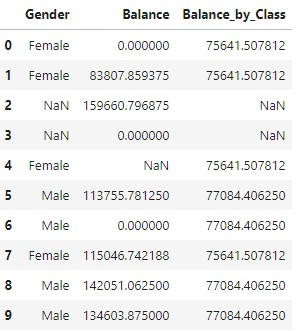df['Balance_imputed'] = np.where(np.isnan(df['Balance']), df['Balance_by_Class'], df['Balance'])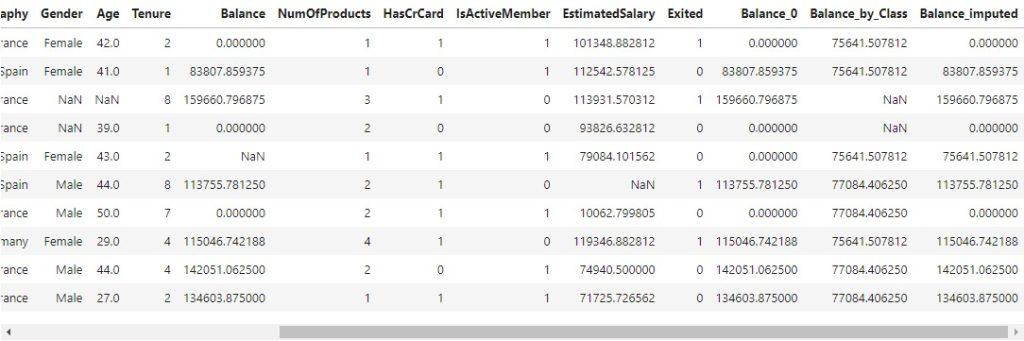## Related Lessons

Interpolation is another useful strategy to impute missing values. But you need to be a bit careful when and how to apply. This is discussed in the following posts.

## Similar Articles

### Logistic Regression – A Complete Tutorial With Examples in RCourse Preview

## Machine Learning A-Z™: Hands-On Python & R In Data Science

### Free Sample Videos:#### Machine Learning A-Z™: Hands-On Python & R In Data Science#### Machine Learning A-Z™: Hands-On Python & R In Data Science#### Machine Learning A-Z™: Hands-On Python & R In Data Science#### Machine Learning A-Z™: Hands-On Python & R In Data Science